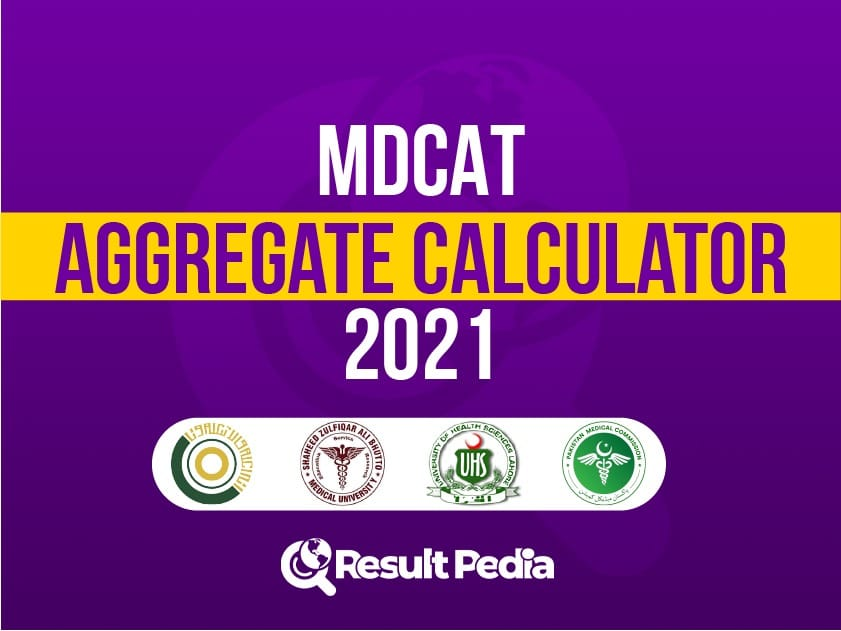Resultpedia# 2023 MDCAT Aggregate Calculator: Find MeritResult Pedia updated its old MDCAT aggregate calculator to help medical students to calculate their MDCAT aggregate. Because it has been a week since the announcement of the MDCAT or NMDCAT( National Medical and Dental College Admission Test) result 2021. But still, most of the students are having difficulty in aggregate calculations, of MDCAT result 2021, to get admission to medical colleges

Other online MDCAT aggregate calculators are available, but the problem is that they are outdated and calculate aggregate based on previous formulas used by medical and dental colleges formerly.

With the latest MDCAT aggregate calculator, 2021 medical students can get their aggregate in no time and according to the new policies of medical colleges.

## Latest features of the MDCAT aggregate calculator

According to the new formula marks of only elective subjects shall be counted while calculating the MDCAT aggregate. Many of the students are confused regarding the formula. Our MDCAT aggregate calculator has made the calculation easier. To calculate the aggregate add the total marks of F.Sc elective subjects instead of putting combined F.Sc numbers constituting all the subjects.

Students can now calculate their NUMS test aggregate with our MBBS aggregate calculator as well.
It is an additional feature with which students can take out aggregate for different medical college admission tests in one place.

With the MDCAT merit calculator, F.Sc medical students can get the aggregate with or without adding their matric marks depending upon the division of grades.

Last but not least, the other important feature of the MDCAT Aggregate calculator 2022 is its accuracy and faster speed.

Recommended Books for MDCAT

## Criteria for calculating MDCAT aggregate

Criteria for selection in medical colleges differ based on the division of marks. Usually, the division criteria involve two basic formulas. The two are:

Numbers obtained in F.Sc or equivalent=50%
Marks obtained in the entrance test =50%

Or

Marks obtained in matric or equivalent=10%
Marks obtained in F.Sc or equivalent=40%
Numbers obtained in F.Sc or equivalent=50%

You can calculate the aggregate for different medical colleges such as NUMS with our aggregate calculator even if the criteria for the division of marks differ.

Start MDCAT preparation